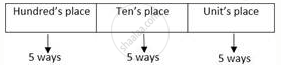# How many three-digit numbers can be formed using the digits 2, 3, 4, 5, 6 if digits can be repeated? - Mathematics and Statistics

Sum

How many three-digit numbers can be formed using the digits 2, 3, 4, 5, 6 if digits can be repeated?

#### Solution

A three-digit number is to be formed from the digits 2, 3, 4, 5, 6 where digits can be repeated.Here, all the places can be filled in 5 ways each.
∴ By using the fundamental principle of multiplication, the total number of three-digit numbers = 5 × 5 × 5 = 125

Concept: Concept of Factorial Function
Is there an error in this question or solution?

#### APPEARS IN

Balbharati Mathematics and Statistics 2 (Commerce) 11th Standard HSC Maharashtra State Board
Chapter 6 Permutations and Combinations
Exercise 6.1 | Q 6 | Page 73